I previously showed an easy way to visualize a regression model that has several continuous explanatory variables: use the SLICEFIT option in the EFFECTPLOT statement in SAS to create a sliced fit plot. The EFFECTPLOT statement is directly supported by the syntax of the GENMOD, LOGISTIC, and ORTHOREG procedures in SAS/STAT. If you are using another SAS regression procedure, you can still visualize multivariate regression models:

• If a procedure supports the STORE statement, you can save the model to an item store and then use the EFFECTPLOT statement in PROC PLM to create a sliced fit plot.
• If a procedure does not support the STORE statement, you can manually create the "slice" of observations and score the model on the slice.

Use PROC PLM to score regression models

Most parametric regression procedures in SAS (GLM, GLIMMIX, MIXED, ...) support the STORE statement, which enables you to save a representation of the model in a SAS item store. The following program creates sample data for 500 patients in a medical study. The call to PROC GLM fits a linear regression model that predicts the level of cholesterol from five explanatory variables. The STORE statement saves the model to an item store named 'GLMModel'. The call to PROC PLM creates a sliced fit plot that shows the predicted values versus the systolic blood pressure for males and females in the study. The explanatory variables that are not shown in the plot are set to reference values by using the AT option in the EFFECTPLOT statement:

```data Heart; /* create example data */ set sashelp.heart(obs=500); where cholesterol < 400; run;   proc glm data=Heart; class Sex Smoking_Status BP_Status; model Cholesterol = Sex Smoking_Status BP_Status /* class vars */ Systolic Weight; /* contin vars */ store GLMModel; /* save the model to an item store */ run;   proc plm restore=GLMModel; /* load the saved model */ effectplot slicefit / at(Smoking_Status='Non-smoker' BP_Status='Normal' Weight=150); /* create the sliced fit plot */ run;```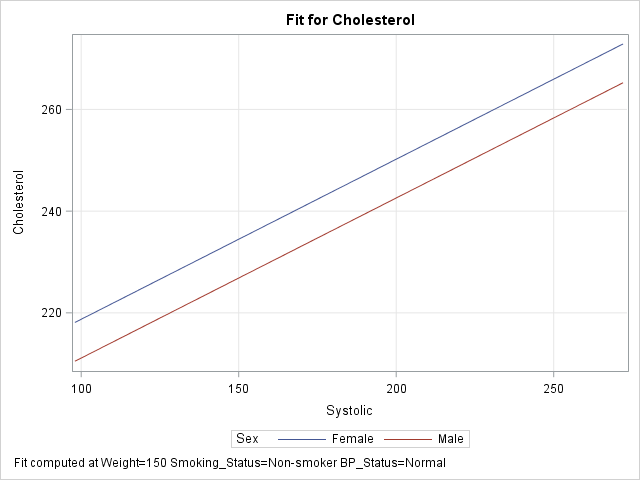The graph shows a sliced fit plot. The footnote states that the lines obtained by slicing through two response surfaces that correspond to (Smoking_Status, BP_Status) = ('Non-smoker', 'Normal') at the value Weight = 150. As shown in the previous article, you can specify multiple values within the AT option to obtain a panel of sliced fit plots.

Create a sliced fit plot manually by using the SCORE statement

The nonparametric regression procedures in SAS (ADAPTIVEREG, GAMPL, LOESS, ...) do not support the STORE statement. Nevertheless, you can create a sliced fit plot using a traditional scoring technique: use the DATA step to create observations in the plane of the slice and score the model on those observations.

There are two ways to score regression models in SAS. The easiest way is to use PROC SCORE, the SCORE statement, or the CODE statement. The following DATA step creates the same "slice" through the space of explanatory variables as was created by using the EFFECTPLOT statement in the previous example. The SCORE statement in the ADAPTIVEREG procedure then fits the model and scores it on the slice. (Technical note: By default, PROC ADAPTIVEREG uses variable selection techniques. For easier comparison with the model from PROC GLM, I used the KEEP= option on the MODEL statement to force the procedure to keep all variables in the model.)

```/* create the scoring observations that define the slice */ data Score; length Sex \$6 Smoking_Status \$17 BP_Status \$7; /* same as for data */ Cholesterol = .; /* set response variable to missing */ Smoking_Status='Non-smoker'; /* set reference levels ("slices") */ BP_Status='Normal'; /* for class vars */ Weight=150; /* and continuous covariates */ do Sex = "Female", "Male"; /* primary class var */ do Systolic = 98 to 272 by 2; /* evenly spaced points for X variable */ output; end; end; run;   proc adaptivereg data=Heart; class Sex Smoking_Status BP_Status; model Cholesterol = Sex Smoking_Status BP_Status Systolic Weight / nomiss /* for comparison with other models, FORCE all variables to be selected */ keep=(Sex Smoking_Status BP_Status Systolic Weight); score data=Score out=ScoreOut Pred; /* score the model on the slice */ run;   proc sgplot data=ScoreOut; series x=Systolic y=Pred / group=Sex; /* create sliced fit plot */ xaxis grid; yaxis grid; run;```

The output, which is not shown, is very similar to the graph in the previous section.

Create a sliced fit plot manually by using the missing value trick

If your regression procedure does not support a SCORE statement, an alternative way to score a model is to use "the missing value trick," which requires appending the scoring data set to the end of the original data. I like to add an indicator variable to make it easier to know which observations are data and which are for scoring. The following statements concatenate the original data and the observations in the slice. It then calls the GAMPL procedure to fit a generalized additive model (GAM) by using penalized likelihood (PL) estimation.

```/* missing value trick: append score data to original data */ data All; set Heart /* data to fit the model */ Score(in=s); /* grid of values on which to score model */ ScoreData=s; /* SCoreData=0 for orig data; =1 for scoring observations */ run;   proc gampl data=All; class Sex Smoking_Status BP_Status; model Cholesterol = Param(Sex Smoking_Status BP_Status) Spline(Systolic Weight); output out=GamOut pred; id ScoreData Sex Systolic; /* include these vars in output data set */ run;   proc sgplot data=GamOut(where=(ScoreData=1)); /* plot only the scoring obs */ series x=Systolic y=Pred / group=Sex; /* create sliced fit plot */ xaxis grid; yaxis grid; run;```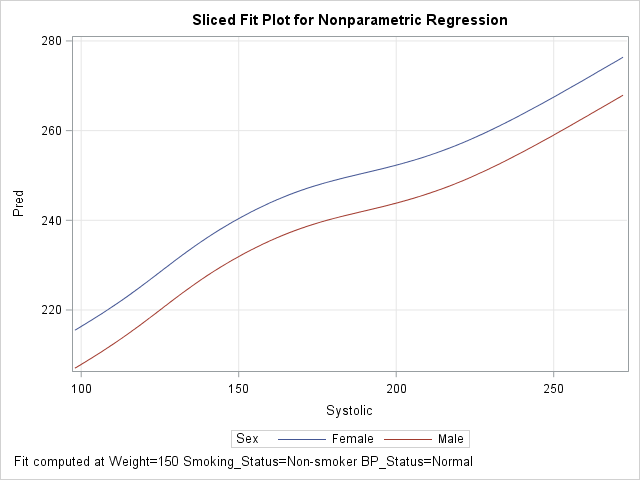The GAMPL procedure does not automatically include all input variables in the output data set; the ID statement specifies the variables that you want to output. The OUTPUT statement produces predicted values for all observations in the ALL data set, but the call to PROC SGPLOT creates the sliced plot by using only the observations for which ScoreData = 1. The output shows the nonparametric regression model from PROC GAMPL.

You can also use the ALL data set to overlay the original data and the sliced fit plot. The details are left as an exercise for the reader.

Summary

The EFFECTPLOT statement provides an easy way to create a sliced fit plot. You can use the EFFECTPLOT statement directly in some regression procedures (such as LOGISTIC and GENMOD) or by using the STORE statement to save the model and PROC PLM to display the graph. For procedures that do not support the STORE statement, you can use the DATA step to create "the slice" (as a scoring data set) and use traditional scoring techniques to evaluate the model on the slice.

The post How to create a sliced fit plot in SAS appeared first on The DO Loop.Slice, slice, baby! You've got to slice, slice, baby!

When you fit a regression model that has multiple explanatory variables, it is a challenge to effectively visualize the predicted values. This article describes how to visualize the regression model by slicing the explanatory variables. In SAS, you can use the SLICEFIT option in the EFFECTPLOT statement visualize a slice of a regression surface.

Why the naive visualization fails

For a regression model that contains one explanatory variable and (optionally) one classification variable, it is easy to visualize the predicted values. Most statistical software packages make it easy to create a "fit plot." For example, the following call to PROC GLM in SAS fits a model to some patients in a heart study:

```data Heart; /* create example data */ set sashelp.heart(obs=500); where cholesterol < 400; run;   ods graphics / attrpriority=none /* groups determine symbols and line patterns */ imagemap tipmax=1500; /* enable tool tips */   /* easy to visualize predicted values for 1 continuous and 1 categorical explanatory variable */ proc glm data=Heart plots=meanplot; /* PLOTS= option supported in many procedures */ class Sex; model Cholesterol = Sex Systolic; quit;```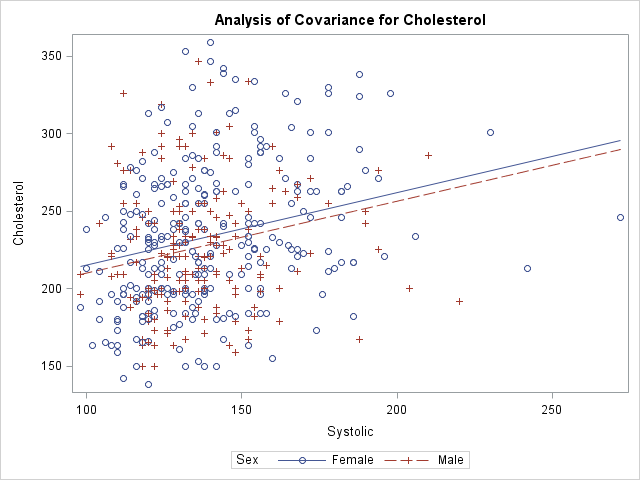The graph shows the observed responses versus the continuous explanatory variable and overlays two curves: one for the predicted values when Sex='Male' and the other when Sex='Female'. Creating this graph is easy because the procedure does all the work.

What happens if you add additional explanatory variables into the model and try to create the same graph? For reasons that will soon be apparent, the procedure will not automatically create the graph when there are additional variables in the model. However, you can use the OUTPUT statement to write the predicted values to a SAS data set and use PROC SGPLOT to create the graph. You will need to sort by the variable that you are plotting on the X axis, as follows:

```proc glm data=Heart; class Sex Smoking_status; model Cholesterol = Sex Smoking_Status /* two classification variables */ Systolic Weight; /* two continuous variables */ output out=GLMOut p=Pred; /* output data set contains predicted values */ quit;   proc sort data=GLMOut; by Systolic Sex; run; /* sort by X variable for graphing */   title "Predicted Values"; proc sgplot data=GLMOut; styleattrs datalinepatterns=(solid solid); scatter x=Systolic y=Cholesterol / group=Sex transparency=0.75; series x=Systolic y=Pred / group=Sex tip=(Smoking_Status Weight); /* add tool tips */ yaxis min=180 max=300; /* zoom in on predicted values */ footnote J=L "Jagged Lines Because Covariates Have Multiple Values"; run;```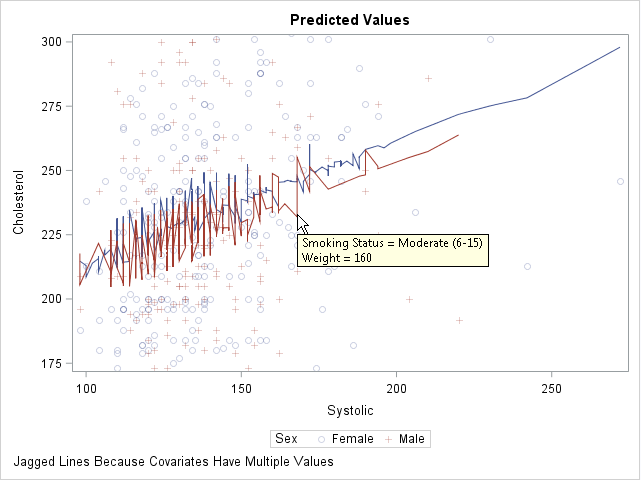This graph looks strange. The regression model is linear, but a plot of the predicted values shows a jagged line for the predicted values. What is going on?

You can use the tool tips feature of the graph to understand why the curves are jagged. If you hover the cursor near a point on the jagged line, the values of the hidden explanatory variables (Weight and Smoking_Status) appear. The graph shows the tool tip at a point that corresponds to a male patient who weighs 160 pounds and who is a moderate smoker. By moving the cursor, you can discover that the previous point along the red line corresponds to a male patient who weighs 155 pounds and is a non-smoker. The subsequent point corresponds to a heavy smoker who weighs 151 pounds.

Because Weight and Smoking_Status were included in the model, the predicted values "jump" up or down as you move along the Systolic axis. Two observations that have similar Systolic values might have very different values for other (hidden) components. Geometrically, this graph displays the projection of the predicted values onto the two-dimensional (Systolic, Cholesterol) plane. To obtain a smooth curve, you must "slice" a response surface rather than project it.

Slice the response surfaces

The predicted values for this model form a set of 10 planes in the three-dimensional space (x, y, z) = (Systolic, Weight, Cholesterol). Each plane is the graph of predicted values for a combination of the 2 genders and 5 levels of smokers. There is one plane is for the ('Male', 'Non-smoker') patients, another for the ('Female', 'Light (1-5)') patients, and so on.

A "slice" through the response surfaces is accomplished by evaluating the model at a particular value of one of the continuous variables. This gives a two-dimensional plot that has 10 lines on it. Because 10 lines might overcrowd the display, it is common to pick a reference value for one of the classification variables and plot only the lines that are indexed by that value. For example, if you choose the reference value Smoking_Status = 'Non-smoker', the plot contains two lines that correspond to ('Male', 'Non-smoker') and ('Female', 'Non-smoker').

This might sound complicated, but SAS provides an easy implementation: the SLICEFIT option in the EFFECTPLOT statement, which is supported in several regression procedures, enables you to specify how you want to slice the surfaces and which combinations of levels you want to display.

By default, the EFFECTPLOT SLICEFIT statement creates a "sliced fit plot" that graphs the response variable versus the first continuous variable and shows the predicted values for each level of the first class variable. "First" is determined by the order in which the variables are listed on the MODEL statement. Other continuous variables are sliced (evaluated) at their mean value; other classification variables are evaluated at their last level.

PROC GLM does not support the EFFECTPLOT statement, but PROC GENMOD does. The following call to PROC GENMOD fits the same model and creates a "sliced fit plot" of the predicted values. The sliced fit plot will show the response variable (Cholesterol) versus the first continuous variable (Systolic) overlaid with predictions for males and females. The value of the Weight variable is set to 151.7, which is the mean value of the sample. The value of the Smoking_Status variable is set to 'Very Heavy (> 25)', which is the last level in alphanumeric order.

```title; footnote; ods graphics / attrpriority=none imagemap=off; proc genmod data=Heart; class Sex Smoking_status; model Cholesterol = Sex Smoking_Status /* classification variables */ Systolic Weight; /* continuous variables */ /* Plot response vs first cont var for each level of first class var */ /* Set other cont vars to MEAN; set other class vars to last level */ effectplot slicefit / obs; /* add scatter plot of observations */ run;```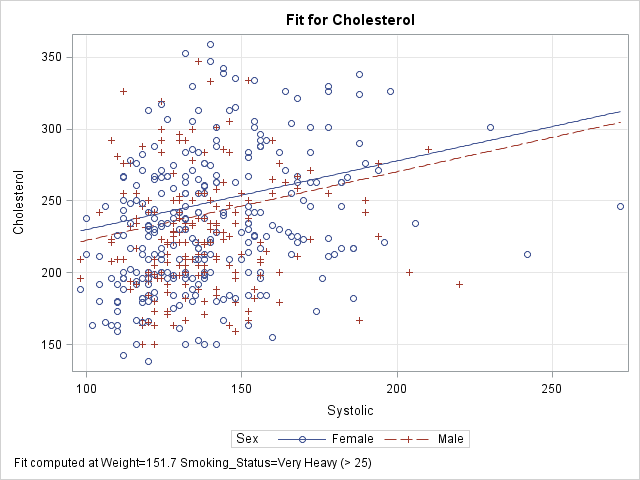The sliced fit plot shows smooth (not jagged) lines because the model is evaluated at constant values of the hidden variables. The values (Weight, Smoking_Status) = (151.7, 'Very Heavy (> 25)') are held constant while the model is evaluated over the range of the Systolic and Sex variables.

Other ways to slice the response surfaces

The SLICEFIT option in the EFFECTPLOT statement supports many suboptions that enable you to control the way that the model is sliced:

• You can plot any two variables, one continuous and one categorical. Use the X= option to specify the continuous variable and the SLICEBY= option to specify the categorical variable.
• You can specify the statistics that are used to slice the continuous covariates. By default the covariates are sliced at their mean values. You can use the AT option to specify the following keywords: MEAN (the default), MIN, MAX, MEDIAN, or MIDRANGE. (Recall that the midrange is the value (min+max)/2.) For class variables, the REF option specifies that the last level be used.
• You can use the AT option to specify particular values for slicing the continuous covariates and class variables.
• You can specify multiple values for the AT option. The EFFECTPLOT statement will create a panel of sliced fit plots, one for each joint combination of specified values.

The following four EFFECTPLOT statements correspond to the four items in the previous list:

```proc genmod data=Heart; class Sex Smoking_status; model Cholesterol = Sex Smoking_Status /* classification variables */ Systolic Weight; /* continuous variables */ /* specify the X and categorical variables */ effectplot slicefit(X=weight sliceby=Smoking_status) / obs;   /* specify statistics used to slice the covariates */ effectplot slicefit / at MIDRANGE /* new default for continuous vars */ REF; /* default for classification vars */   /* specify explicit values of the covariates */ effectplot slicefit / at(Weight=150 Smoking_Status='Non-smoker');   /* specify multiple values of the covariates to get a panel */ effectplot slicefit / at(Weight=150 200 Smoking_Status='Non-smoker' 'Heavy (16-25)'); quit;```

To save space, only the last sliced fit plot (the panel) is shown below. I have linked to the other three plots: the plot of Weight and Smoking_Status, the plot at midrange, and the plot at specified values.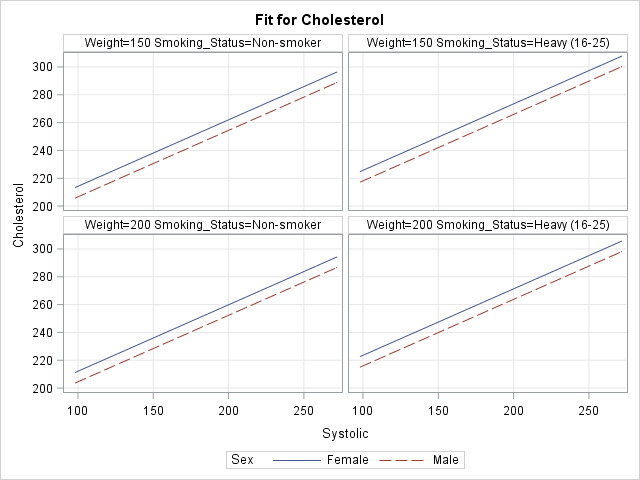In summary, you can use the SLICEFIT option in the EFFECTPLOT statement in SAS to visualize regression models that contain many explanatory variables. The AT option enables you to specify values for the covariates. The resulting graph displays a slice through the response surface.

The EFFECTPLOT statement is also available in PROC PLM. PROC PLM enables you to visualize a model that has been saved to an item store. The OBS option (which overlays the predicted values and a scatter plot) is not available in PROC PLM because the item store does not include the observations.

The post Visualize multivariate regression models by slicing continuous variables appeared first on The DO Loop.In a previous article, I showed how to use SAS to perform mean imputation. However, there are three problems with using mean-imputed variables in statistical analyses:

• Mean imputation reduces the variance of the imputed variables.
• Mean imputation shrinks standard errors, which invalidates most hypothesis tests and the calculation of confidence interval.
• Mean imputation does not preserve relationships between variables such as correlations.

This article explores these issues in more detail. The example data set (called IMPUTED) was created in the previous article. It is a modification of the Sashelp.Class data in which heights of seven students are assigned missing value. Mean imputation replaces those seven value with the mean of the observed values. The Orig_Height variable contains the original (missing) values; the Height variable contains the imputed values.

Mean imputation reduces variance

The following call to PROC MEANS computes simple descriptive statistics for the original and imputed variables. The statistics for the original variable are computed by using listwise deletion, which means that missing observations are dropped from the analysis.

```proc means data=Imputed ndec=2 N NMiss Mean StdErr StdDev; var Orig_Height Height; /* compare stats original and imputed vars */ run;```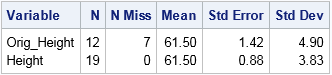The mean-imputed variable (Height) has the same mean as the original variable (Orig_Height). This is always the case for mean-imputed data. However, notice that the standard deviation (hence, variance) of the imputed variable is smaller. You can see this by overlaying the distributions of the original and imputed variables, as follows:

```title "Comparison of Original and Imputed Data"; proc sgplot data=Imputed; xaxis label="Height" values=(52 to 70 by 4 61.5) valueshint; yaxis grid; histogram Height / scale=count binstart=52 binwidth=4 legendlabel="Imputed"; histogram Orig_Height / scale=count binstart=52 binwidth=4 legendlabel="Original" transparency=0.5; refline 61.5 / axis=x lineattrs=(color=black) label="Mean"; /* mean value */ run;```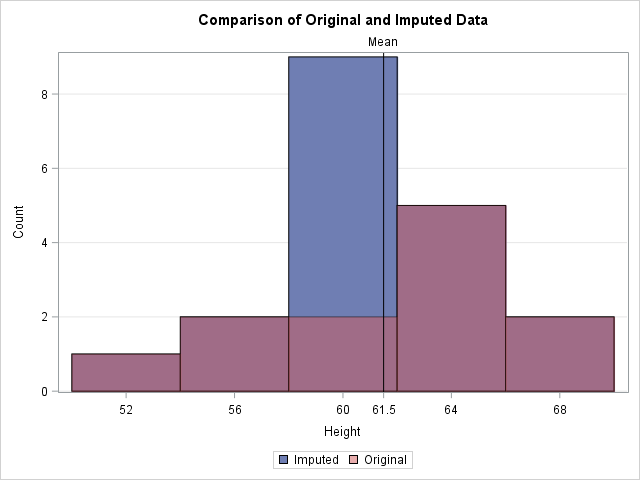In the graph, the reddish bars show the distribution of the observed values. Behind those bars is a second histogram (in blue) that shows the distribution of the imputed data. The only bar of the second histogram that is visible is the one that contains the sample mean. The graph emphasizes the fact that all imputed values are equal to the mean. This reduces the variance of the imputed variable because none of the imputed values contribute to the variance (which is based on deviations from the mean). Thus the variance of the mean-imputed variable is always smaller than the variance of the original variable.

Mean imputation shrinks standard errors and confidence intervals

The previous section shows that the imputed variable always has a smaller variance than original variable. The estimated variance is used to compute many other statistics, which are also shrunk. For example, the following statistics are shrunk for the imputed variable as compared to the original variable:

1. The standard error of the mean, as shown in the previous output from PROC MEANS.
2. The confidence intervals that are based on mean-imputed data will be shorter.
3. The standard t test for a mean uses the standard error to compute a p-value for the null hypothesis that the population mean equals some value. If the standard error is shrunk by mean imputation, then the standard one-sample t test is not valid and the p-value is too small. You will potentially reject a null hypotheses that might be true (a Type I error).

Mean imputation distorts relationships between variables

The previous sections emphasized how mean imputation affects univariate statistics. But mean imputation also distorts multivariate relationships and affects statistics such as correlation. For example, the following call to PROC CORR computes the correlation between the Orig_Height variable and the Weight and Age variables. It also computes the correlations for the mean-imputed variable (Height). The output shows that the imputed variable has much smaller correlations with Weight and Age because single imputation does not try to preserve multivariate relationships.

```proc corr data=Imputed noprob; var Weight Age; with Orig_Height Height; run;```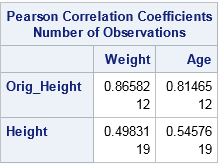In a similar way, a linear regression that attempts to predict Weight by height is corrupted by the replacement of missing values with mean values. For one-variable linear regression, it is easy to show that the estimates of the slope are unchanged by mean imputation, but the intercept estimates can be different. For these data, the least-squares estimate of the slope is 2.96. The intercept estimate for the original data is -90 whereas the intercept for the imputed variable is -82. The following call to PROC SGPLOT shows these estimates graphically:

```ods graphics / attrpriority=NONE; title "Simple Linear Regression with Mean Imputation"; proc sgplot data=Imputed; styleattrs datasymbols=(Circle X); reg x=Orig_Height y=Weight / nomarkers curvelabel="Original"; reg x=Height y=Weight / nomarkers curvelabel="Imputed"; scatter x=Height y=Weight / group=Replaced; xaxis grid; yaxis grid; run;```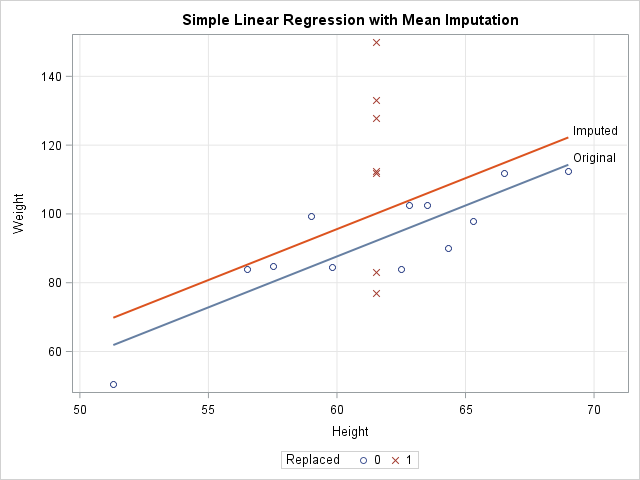The graph shows that the model that uses the original data (the blue line) predicts lower values of Weight than the model that uses the imputed heights (the red line). The scatter plot shows why. The model for the original data uses only 12 observations, which are displayed as blue circles. The predicted value of Weight for the mean height is about 92. The seven imputed values are shown as red X's for which the Height is 61.5. The average Weight for these observations is greater than 92, so the seven observations bias the computation and "pull up" the regression line. For different data, the imputed model might "pull down" the predictions. It depends on the average response for the imputed observations.

Conclusions

Although imputing missing values by using the mean is a popular imputation technique, there are serious problems with mean imputation. The variance of a mean-imputed variable is always biased downward from the variance of the un-imputed variable. This bias affects standard errors, confidence intervals, and other inferential statistics. Experts agree that mean imputation should be avoided when possible (Allison (2009), Horton and Kleinman (2007)).

So what alternatives are there? That question has been the topic of many books and papers. Paul Allison (2009) suggests either maximum likelihood estimation or multiple imputation methods, both of which try to preserve relationships between variables and the inherent variability of the data. In SAS, PROC MI and MIANALYZE work with other SAS/STAT procedures to apply these methods to missing data. You can see the list of procedures that handle missing data in SAS. For more information about the alternatives to single imputation, the following references are good places to start:

The post 3 problems with mean imputation appeared first on The DO Loop.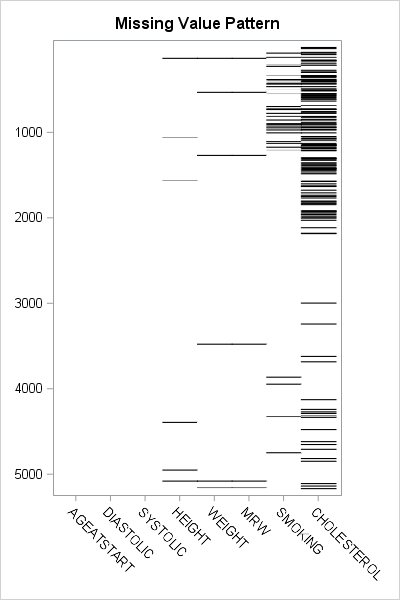Missing values present challenges for the statistical analyst and data scientist. Many modeling techniques (such as regression) exclude observations that contain missing values, which can reduce the sample size and reduce the power of a statistical analysis. Before you try to deal with missing values in an analysis (for example, by using multiple imputation), you need to understand which variables contain the missing values and to examine the patterns of missing values. I have previously written about how to use SAS to do the following:

An example of a visualization of a missing value pattern is shown to the right. The heat map shows the missing data for eight variables and 5209 observations in the Sashelp.Heart data set. It is essentially the heat map of a binary matrix where 1 indicates nonmissing values (white) and 0 indicates missing values (black).

In this article, I present a bar chart that helps to visualize the "Missing Data Patterns" table from PROC MI in SAS/STAT software. I also show two SAS tricks:

The pattern of missing data

The MI procedure can perform multiple imputation of missing data, but it also can be used as a diagnostic tool to group observations according to the pattern of missing data. You can use the NIMPUTE=0 option to display the pattern of missing value. By default, the "Missing Data Patterns" table is very wide because it includes the group means for each variable. However, you can use the DISPLAYPATTERN=NOMEANS option (SAS9.4M5) to suppress the group means, as follows:

```%let Vars = AgeAtStart Height Weight Diastolic Systolic MRW Smoking Cholesterol; %let numVars = %sysfunc(countw(&Vars)); /* &numVars = 8 for this example */   proc mi data=Sashelp.Heart nimpute=0 displaypattern=nomeans; /* SAS 9.4M5 option */ var &Vars; ods output MissPattern=Pattern; /* output missing value pattern to data set */ run;```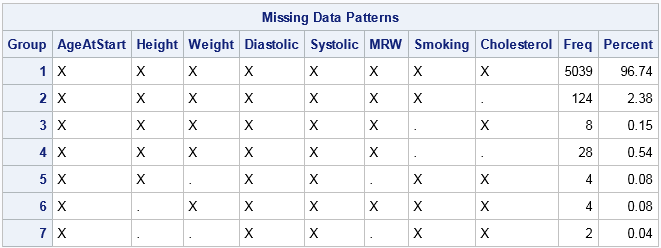In the table, an X indicates that a variable does not contain any missing values, whereas a dot (.) indicates that a variable contains missing values. The table shows that Group 1 consists of about 5000 observations that are complete. Groups 2, 3, and 6 contains observations for which exactly one variable contains missing values. Groups 4 and 5 contain observations for which two variables are jointly missing. Group 7 contains observations for which three variables are missing. You can see that the size of the groups vary widely. Some patterns of missing value appear only a few times, whereas others appear much more often.

By inspecting the table, you can determine which combinations of variables are missing for each group. However, imagine a dataset that has 20 variables. The "Missing Data Patterns" table for 20 variables would be very wide, and it would be cumbersome to determine which combination of variables are jointly missing. The next section condenses the table into a smaller format and then creates a graph to summarize the pattern of missing values.

Shorten the labels for the missing data patterns

The information in the "Missing Data Patterns" table can be condensed. I saw the following idea in Chapter 10 of Gerhard Svolba's Data Quality for Analytics Using SAS, who credits the idea to a display that appears in JMP software. (Svolba does not use PROC MI, but defines a macro that you can download from the book's website.)

The table is wide because there is a column for every variable in the analysis. But the information in those columns is binary: for the i_th group does the j_th variable have a missing value? If the analysis involves k variables, you can replace the k columns by a single binary string that has k digits. The j_th digit in the string indicates whether the j_th variable has a missing value.

In the preceding analysis, the ODS OUTPUT statement wrote the "Missing Data Patterns" table to a data set. The following SAS DATA step reads the data and constructs a binary string of length k from the k character variables in the data. The binary string is formed by using the CATT function to concatenate the 'X' and '.' values in the table. The TRANSLATE function then converts those characters to '0' and '1' characters. The DATA step also computes the NumMiss variable, which counts the number of variables that have missing values for each row:

```data Miss; set Pattern; array vars[*] _CHARACTER_; /* or use &Vars */ length Pattern \$&numVars.; /* length = number of variables in array */ Pattern = translate(catt(of vars[*]), '01', 'X.'); /* Ex: 00100100 */ NumMiss = countc(pattern, '1'); run;   proc print data=Miss noobs; var Group Pattern NumMiss Freq; run;```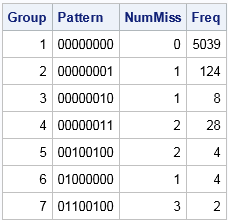The table shows that the eight columns for the analysis variables have been replaced by a single column that displays an eight-character binary string. For Group 1, the string is all zeros, which indicates that no variable has missing values. For Group 2, the binary string contains all zeros except for a 1 in the last position. This means that Group 1 is the set of observations for which the last variable contains a missing value. Similarly, Group 7 is the set of observations for which the second, third, and sixth variables contain a missing value. Notice that this table does not provide the names of the variables. If you cannot remember the name of the second, third, and sixth variables, you need to look them up in the VARS macro variable.

Create a bar chart that has a logarithmic scale

The condensed version of the "Missing Data Patterns" table is suitable to graph as a bar chart. However, for these data the size of the groups vary widely. Consequently, you might want to plot the frequencies of the groups on a logarithmic scale. (Or you might not! There is considerable debate about whether you should display a bar chart that has a logarithmic axis. For a discussion and alternatives, see Sanjay's article "Graphs with log axis." My opinion is that a log axis is fine to use for a technical audience such as statisticians.)

By default, you cannot use a logarithmic scale on a bar chart because the baseline for the vertical axis (the frequency or count axis) starts at 0 and the LOG function is not defined at 0. If you have count data and no category has zero counts, then you can set the baseline of the graph to 1. The bars then indicate the log-frequency of the counts. The following call to PROC SGPLOT creates the bar chart and a marginal table:

```title "Pattern of Missing Values"; proc sgplot data=Miss; hbar Pattern /response=Freq datalabel baseline=1; /* set BASELINE=1 for log scale */ yaxistable NumMiss / valuejustify=left label="Num Miss" valueattrs=GraphValueText labelattrs=GraphLabelText; /* use same attributes as axis */ yaxis labelposition=top; xaxis grid type=log logbase=10 label="Frequency (log10 scale)"; run;```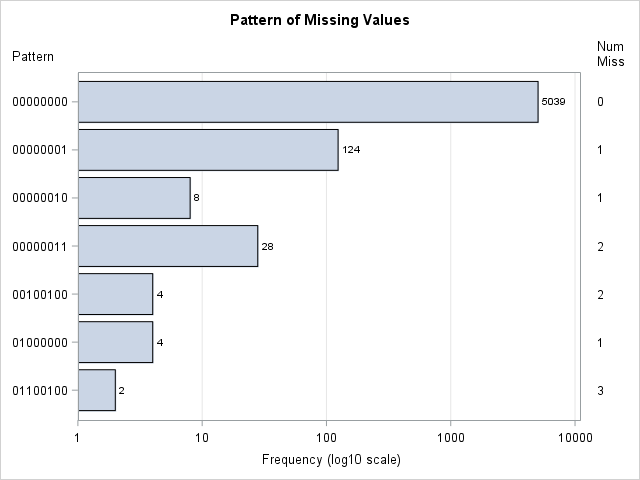This graph displays the counts of the number of observations in each pattern group. The labels on the Y axis indicate which variables have missing data. The values in the right margin indicate how many variables have missing data. If you are opposed to drawing bar charts on a logarithmic axis, you can use one of Sanjay's alternative visualizations.

In summary, you can create a bar chart that visualizes the number of observations that have a similar pattern of missing values. The summarization comes from PROC MI in SAS, which has a new DISPLAYPATTERN=NOMEANS option. A short DATA step can create a binary string for each group. That string can be used to indicate the missing data pattern in each group. If the groups vary widely in size, you can use a logarithmic axis to display the data. To create a bar chart on a logarithmic scale in SAS, set BASELINE=1 in the HBAR statement and use TYPE=LOG on the XAXIS statement in PROC SGPLOT.

The post Visualize patterns of missing values appeared first on The DO Loop.Missing values present challenges for the statistical analyst and data scientist. Many modeling techniques (such as regression) exclude observations that contain missing values, which can reduce the sample size and reduce the power of a statistical analysis. Before you try to deal with missing values in an analysis (for example, by using multiple imputation), you need to understand which variables contain the missing values and to examine the patterns of missing values. I have previously written about how to use SAS to do the following:

An example of a visualization of a missing value pattern is shown to the right. The heat map shows the missing data for eight variables and 5209 observations in the Sashelp.Heart data set. It is essentially the heat map of a binary matrix where 1 indicates nonmissing values (white) and 0 indicates missing values (black).

In this article, I present a bar chart that helps to visualize the "Missing Data Patterns" table from PROC MI in SAS/STAT software. I also show two SAS tricks:

The pattern of missing data

The MI procedure can perform multiple imputation of missing data, but it also can be used as a diagnostic tool to group observations according to the pattern of missing data. You can use the NIMPUTE=0 option to display the pattern of missing value. By default, the "Missing Data Patterns" table is very wide because it includes the group means for each variable. However, you can use the DISPLAYPATTERN=NOMEANS option (SAS9.4M5) to suppress the group means, as follows:

```%let Vars = AgeAtStart Height Weight Diastolic Systolic MRW Smoking Cholesterol; %let numVars = %sysfunc(countw(&Vars)); /* &numVars = 8 for this example */   proc mi data=Sashelp.Heart nimpute=0 displaypattern=nomeans; /* SAS 9.4M5 option */ var &Vars; ods output MissPattern=Pattern; /* output missing value pattern to data set */ run;```In the table, an X indicates that a variable does not contain any missing values, whereas a dot (.) indicates that a variable contains missing values. The table shows that Group 1 consists of about 5000 observations that are complete. Groups 2, 3, and 6 contains observations for which exactly one variable contains missing values. Groups 4 and 5 contain observations for which two variables are jointly missing. Group 7 contains observations for which three variables are missing. You can see that the size of the groups vary widely. Some patterns of missing value appear only a few times, whereas others appear much more often.

By inspecting the table, you can determine which combinations of variables are missing for each group. However, imagine a dataset that has 20 variables. The "Missing Data Patterns" table for 20 variables would be very wide, and it would be cumbersome to determine which combination of variables are jointly missing. The next section condenses the table into a smaller format and then creates a graph to summarize the pattern of missing values.

Shorten the labels for the missing data patterns

The information in the "Missing Data Patterns" table can be condensed. I saw the following idea in Chapter 10 of Gerhard Svolba's Data Quality for Analytics Using SAS, who credits the idea to a display that appears in JMP software. (Svolba does not use PROC MI, but defines a macro that you can download from the book's website.)

The table is wide because there is a column for every variable in the analysis. But the information in those columns is binary: for the i_th group does the j_th variable have a missing value? If the analysis involves k variables, you can replace the k columns by a single binary string that has k digits. The j_th digit in the string indicates whether the j_th variable has a missing value.

In the preceding analysis, the ODS OUTPUT statement wrote the "Missing Data Patterns" table to a data set. The following SAS DATA step reads the data and constructs a binary string of length k from the k character variables in the data. The binary string is formed by using the CATT function to concatenate the 'X' and '.' values in the table. The TRANSLATE function then converts those characters to '0' and '1' characters. The DATA step also computes the NumMiss variable, which counts the number of variables that have missing values for each row:

```data Miss; set Pattern; array vars[*] _CHARACTER_; /* or use &Vars */ length Pattern \$&numVars.; /* length = number of variables in array */ Pattern = translate(catt(of vars[*]), '01', 'X.'); /* Ex: 00100100 */ NumMiss = countc(pattern, '1'); run;   proc print data=Miss noobs; var Group Pattern NumMiss Freq; run;```The table shows that the eight columns for the analysis variables have been replaced by a single column that displays an eight-character binary string. For Group 1, the string is all zeros, which indicates that no variable has missing values. For Group 2, the binary string contains all zeros except for a 1 in the last position. This means that Group 1 is the set of observations for which the last variable contains a missing value. Similarly, Group 7 is the set of observations for which the second, third, and sixth variables contain a missing value. Notice that this table does not provide the names of the variables. If you cannot remember the name of the second, third, and sixth variables, you need to look them up in the VARS macro variable.

Create a bar chart that has a logarithmic scale

The condensed version of the "Missing Data Patterns" table is suitable to graph as a bar chart. However, for these data the size of the groups vary widely. Consequently, you might want to plot the frequencies of the groups on a logarithmic scale. (Or you might not! There is considerable debate about whether you should display a bar chart that has a logarithmic axis. For a discussion and alternatives, see Sanjay's article "Graphs with log axis." My opinion is that a log axis is fine to use for a technical audience such as statisticians.)

By default, you cannot use a logarithmic scale on a bar chart because the baseline for the vertical axis (the frequency or count axis) starts at 0 and the LOG function is not defined at 0. If you have count data and no category has zero counts, then you can set the baseline of the graph to 1. The bars then indicate the log-frequency of the counts. The following call to PROC SGPLOT creates the bar chart and a marginal table:

```title "Pattern of Missing Values"; proc sgplot data=Miss; hbar Pattern /response=Freq datalabel baseline=1; /* set BASELINE=1 for log scale */ yaxistable NumMiss / valuejustify=left label="Num Miss" valueattrs=GraphValueText labelattrs=GraphLabelText; /* use same attributes as axis */ yaxis labelposition=top; xaxis grid type=log logbase=10 label="Frequency (log10 scale)"; run;```This graph displays the counts of the number of observations in each pattern group. The labels on the Y axis indicate which variables have missing data. The values in the right margin indicate how many variables have missing data. If you are opposed to drawing bar charts on a logarithmic axis, you can use one of Sanjay's alternative visualizations.

In summary, you can create a bar chart that visualizes the number of observations that have a similar pattern of missing values. The summarization comes from PROC MI in SAS, which has a new DISPLAYPATTERN=NOMEANS option. A short DATA step can create a binary string for each group. That string can be used to indicate the missing data pattern in each group. If the groups vary widely in size, you can use a logarithmic axis to display the data. To create a bar chart on a logarithmic scale in SAS, set BASELINE=1 in the HBAR statement and use TYPE=LOG on the XAXIS statement in PROC SGPLOT.

The post Visualize patterns of missing values appeared first on The DO Loop.In a previous article, I showed how to compute a principal component regression in SAS. Recall that principal component regression is a technique for handling near collinearities among the regression variables in a linear regression. The PCR algorithm in most statistical software is more correctly called "incomplete" PCR because it uses only a subset of the principal components. Incomplete PCR means that you compute the principal components for the explanatory variables, keep only the first k principal components (which explain most of the variance among the regressors), and regress the response variable onto those k components.

The principal components that are dropped correspond to the near collinearities. Consequently, the standard errors of the parameter estimates are reduced, although the tradeoff is that the estimates are biased, and "the bias increases as more [principal components]are droppped" (Jackson, p. 276).

Some arguments in this article are from J. E. Jackson's excellent book, A User's Guide to Principal Components, (1991, pp. 271–278). Jackson introduces PCR and then immediately cautions against using it (p. 271). He writes that PCR "is a widely used technique," but "it also has some serious drawbacks." Let's examine the advantages and disadvantages of principal component regression.

Principal component regression is a popular and widely used method. Advantages of PCR include the following:

• PCR can perform regression when the explanatory variables are highly correlated or even collinear.
• PCR is intuitive: you replace the basis {X1, X2, ..., Xp} with an orthogonal basis of principal components, drop the components that do not explain much variance, and regress the response onto the remaining components.
• PCR is automatic: The only decision you need to make is how many principal components to keep.
• The principal components that are dropped give insight into which linear combinations of variables are responsible for the collinearities.
• PCR has a discrete parameter, namely the number of components kept. This parameter is very interpretable in terms of geometry (linear dimensions kept) and in terms of linear algebra (low-rank approximations).
• You can run PCR when there are more variables than observations (wide data).

Drawbacks of principal component regression

The algorithm that is currently known as PCR is actually a misinterpretation of the original ideas behind PCR (Jolliffe, 1982, p. 201). When Kendall and Hotelling first proposed PCR in the 1950s, they proposed "complete" PCR, which means replacing the original variables by all the principal components, thereby stabilizing the numerical computations. Which principal components are included in the final model is determined by looking at the significance of the parameter estimates. By the early 1980s, the term PCR had changed to mean "incomplete PCR."

The primary argument against using (incomplete) principal component regression can be summarized in a single sentence: Principal component regression does not consider the response variable when deciding which principal components to drop. The decision to drop components is based only on the magnitude of the variance of the components.

There is no a priori reason to believe that the principal components with the largest variance are the components that best predict the response. In fact, it is trivial to construct an artificial example in which the best predictor is the last component, which will surely be dropped from the analysis. (Just define the response to be the last principal component!) More damning, Jolliffe (1982, p. 302) presents four examples from published papers that advocate PCR, and he shows that some of the low-variance components (which were dropped) have greater predictive power than the high-variance components that were kept. Jolliffe concludes that "it is not necessary to find obscure or bizarre data in order for the last few principal components to be important in principal component regression. Rather it seems that such examples may be rather common in practice."

There is a hybrid version of PCR that enables you to use cross validation and the predicted residual sum of squares (PRESS) criterion to select how many components to keep. (In SAS, the syntax is proc pls method=PCR cv=one cvtest(stat=press).) Although this partially addresses the issue by including the response variable in the selection of components, it is still the case that the first k components are selected and the last p – k are dropped. The method never keeps the first, third, and sixth components, for example.

Alternatives to principal component regression

Some alternatives to principal component regression include the following:

• Ridge regression: In ridge regression, a diagonal matrix is added to the X`X matrix so that it becomes better conditioned. This results in biased parameter estimates. You can read an explanation of ridge regression and how to compute it by using PROC REG in SAS.
• Complete PCR: As mentioned previously, use the PCs as the variables and keep the components whose parameter estimates are significant.
• Complete PCR with variable selection: Use the PCs as the variables and use the variable-selection techniques to decide which components to retain. However, if your primary goal is variable reduction, then use variable-selection techniques on the original variables.
• Partial Least Squares (PLS): Partial least square regression is similar to PCR in that both select components that explain the most variance in the model. The difference is that PLS incorporates the response variable. That is, the components that are produced are those that explain the most variance in the explanatory AND response variables. In SAS, you can compute a PLS regression by using PROC PLS with METHOD=PLS or METHOD=SIMPLS. You will probably also want to use the CV and CVTEST options.

Summary

In summary, principal component regression is a technique for computing regressions when the explanatory variables are highly correlated. It has several advantages, but the main drawback of PCR is that the decision about how many principal components to keep does not depend on the response variable. Consequently, some of the variables that you keep might not be strong predictors of the response, and some of the components that you drop might be excellent predictors. A good alternative is partial least squares regression, which I recommend. In SAS, you can run partial least squares regression by using PROC PLS with METHOD=PLS.

References

The post Should you use principal component regression? appeared first on The DO Loop.In a previous article, I discussed the lines plot for multiple comparisons of means. Another graph that is frequently used for multiple comparisons is the diffogram, which indicates whether the pairwise differences between means of groups are statistically significant. This article discusses how to interpret a diffogram. Two related plots are also discussed.

How to create a diffogram in SAS

The diffogram (also called a mean-mean scatter diagram) is automatically created when you use the PDIFF=ALL option on the LSMEANS statement in several SAS/STAT regression procedures such as PROC GLM and PROC GLMMIX, assuming that you have enabled ODS graphics. The documentation for PROC GLM contains an example that uses data about the chemical composition of shards of pottery from four archaeological sites in Great Britain. Researchers want to determine which sites have shards that are chemically similar. The data are contained in the pottery data set. The following call to PROC GLM performs an ANOVA of the calcium oxide in the pottery at the sites. The PDIFF=ALL option requests an analysis of all pairwise comparisons between the LS-means of calcium oxide for the different sites. The ADJUST=TUKEY option is one way to adjust the confidence intervals for multiple comparisons.

```ods graphics on; proc glm data=pottery plots(only)=( diffplot(center) /* diffogram */ meanplot(cl ascending) ); /* plot of means and CIs */ label Ca = "Calcium Oxide (%)"; class Site; model Ca = Site; lsmeans Site / pdiff=all cl adjust=tukey; /* all pairwise comparisons of means w/ adjusted CL */ ods output LSMeanDiffCL=MeanDiff; /* optional: save mean differences and CIs */ quit;```

Two graphs are requested: the diffogram (or "diffplot") and a "mean plot" that shows the group means and 95% confidence intervals. The ODS OUTPUT statement creates a data set from a table that contains the mean differences between pairs of groups, along with 95% confidence intervals for the differences. You can use that information to construct a plot of the mean differences, as shown later in this article.

How to interpret a diffogram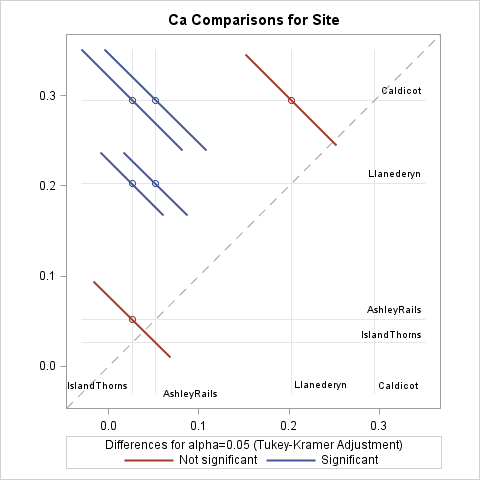The diffogram, which is shown to the right (click to enlarge), is my favorite graph for multiple comparisons of means. Every diffogram displays a diagonal reference line that has unit slope. Horizontal and vertical reference lines are placed along the axes at the location of the means of the groups. For these data, there are four vertical and four horizontal reference lines. At the intersection of most reference lines there is a small colored line segment. These segments indicate which of the 4(4-1)/2 = 6 pairwise comparisons of means are significant.

Let's start at the top of the diffogram. The mean for the Caldecot site is about 0.3, as shown by a horizontal reference line near Y=0.3. On the reference line for Caldecot are three line segments that are centered at the mean values for the other groups, which are (from left to right) IslandThorns, AshleyRails, and Llanederyn. The first two line segments (in blue) do not intersect the dashed diagonal reference line, which indicates that the means for the (IslandThorns, Caldecot) and (AshleyRails, Caldecot) pairs are significantly different. The third line segment (in red) intersects the diagonal reference line, which indicates that the (Llanederyn, Caldecot) comparison is not significant.

Similarly, the mean for the Llanederyn site is about 0.2, as shown by a horizontal reference line near Y=0.2. On the reference line for Llanederyn are two line segments, which represent the (IslandThorns, Llanederyn) and (AshleyRails, Llanederyn) comparisons. Neither line segment intersects the diagonal reference line, which indicates that the means for those pairs are significantly different.

Lastly, the mean for the AshleyRails site is about 0.05, as shown by a horizontal reference line near Y=0.05. The line segment on that reference line represents the (IslandThorns, AshleyRails) comparison. It intersects the diagonal reference line, which indicates that those means are not significantly different.

The colors for the significant/insignificant pairs depends on the ODS style, but it is easy to remember the simple rule: if a line segment intersects the diagonal reference line, then the corresponding group means are not significantly different. A mnemonic is "Intersect? Insignificant!"

The mean plot with confidence interval: Use wisely!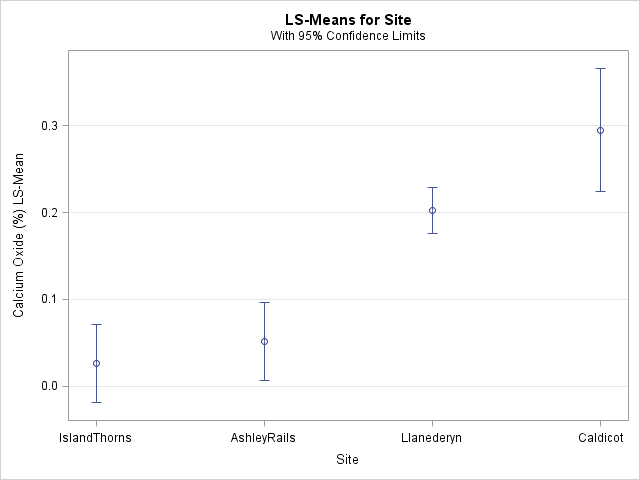The previous section shows how to use the diffogram to visually determine which pairs of means are significantly different. This section reminds you that you should not try to use the mean plot (shown at the right) for making those inferences.

I have seen presentations in which the speaker erroneously claims that "the means of these groups are significantly different because their 95% confidence intervals do not overlap." That is not a correct inference. In general, the overlap (or lack thereof) between two (1 – α)100% confidence intervals does not give sufficient information about whether the difference between the means is significant at the α level. In particular, you can construct examples where

• Two confidence intervals overlap, but the difference of means is significant. (See Figure 2 in High (2014).)
• Two confidence intervals do not overlap, but the difference of means is not significant.

The reason is twofold. First, the confidence intervals in the plot are constructed by using the sample sizes and standard deviations for each group, whereas tests for the difference between the means are constructed by using pooled standard deviations and sample sizes. Second, if you are making multiple comparisons, you need to adjust the widths of the intervals to accommodate the multiple (simultaneous) inferences.

"But Rick," you might say, "in the mean plot for these data, the Llanederyn and Caldecot confidence intervals overlap. The intervals for IslandThorns and AshleyRails also overlap. And these are exactly the two pairs that are not significantly different, as shown by the diffogram!" Yes, that is true for these data, but it is not true in general. Use the diffogram, not the means plot, to visualize multiple comparisons of means.

Plot the pairwise difference of means and confidence intervals

In addition to the diffogram, you can visualize comparisons of means by plotting the confidence intervals for the pairwise mean differences. Those intervals that contain 0 represent insignificant differences. Recall that the call to PROC GLM included an ODS output statement that created a data set (MeanDiff) that contains the mean differences. The following DATA step constructs labels for each pair and computes whether each pairwise difference is significant:

```data Intervals; length Pair \$28.; length S \$16.; set MeanDiff; /* The next line is data dependent. For class variable 'C', concatenate C and _C variables */ Pair = catx(' - ', Site, _Site); Significant = (0 < LowerCL | UpperCL < 0); /* is 0 in interior of interval? */ S = ifc(Significant, "Significant", "Not significant"); run;   title "Pairwise Difference of LSMeans (Tukey Adjustment)"; title2 "95% Confidence Intervals of Mean Difference"; footnote J=L "Pairs Whose Intervals Contain 0 Are Not Significantly Different"; proc sgplot data=Intervals; scatter y=Pair x=Difference / group=S name="CIs" xerrorlower=LowerCL xerrorupper=UpperCL; refline 0 / axis=x; yaxis reverse colorbands=odd display=(nolabel) offsetmin=0.06 offsetmax=0.06; keylegend "CIs" / sortorder=ascending; run;```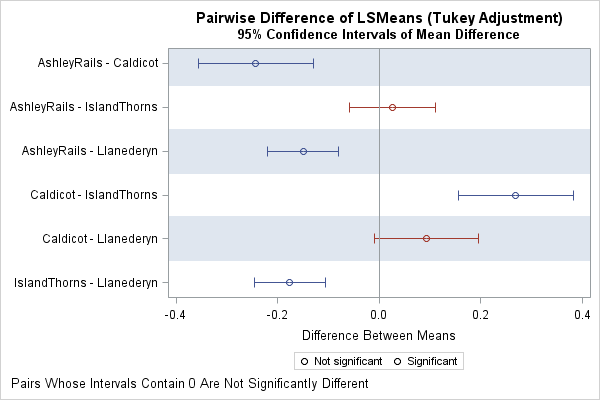The resulting graph is shown to the right. For each pair of groups, the graph shows an estimate for the difference of means and the Tukey-adjusted 95% confidence intervals for the difference. Intervals that contain 0 indicate that the difference of means is not significant. Intervals that do not contain 0 indicate significant differences.

Although the diffogram and the difference-of-means plot provide the same information, I prefer the diffogram because it shows the values for the means rather than for the mean differences. Furthermore, the height of the difference-of-means plot needs to be increased as more groups are added (there are k(k-1)/2 rows for k groups), whereas the diffogram can accommodate a moderate number of groups without being rescaled. On the other hand, it can be difficult to read the reference lines in the diffogram when there are many groups, especially if some of the groups have similar means.

For more about multiple comparisons tests and how to visualize the differences between means, see the following references:

The post The diffogram and other graphs for multiple comparisons of means appeared first on The DO Loop.Last week Warren Kuhfeld wrote about a graph called the "lines plot" that is produced by SAS/STAT procedures in SAS 9.4M5. (Notice that the "lines plot" has an 's'; it is not a line plot!) The lines plot is produced as part of an analysis that performs multiple comparisons of means. Although the graphical version of the lines plot is new in SAS 9.4M5, the underlying analysis has been available in SAS for decades. If you have an earlier version of SAS, the analysis is presented as a table rather than as a graph.

Warren's focus was on the plot itself, with an emphasis on how to create it. However, the plot is also interesting for the statistical information it provides. This article discusses how to interpret the lines plot in a multiple comparisons of means analysis.

The lines plot in SAS

You can use the LINES option in the LSMEANS statement to request a lines plot in SAS 9.4M5. The following data are taken from Multiple Comparisons and Multiple Tests (p. 42-53 of the First Edition). Researchers are studying the effectiveness of five weight-loss diets, denoted by A, B, C, D, and E. Ten male subjects are randomly assigned to each method. After a fixed length of time, the weight loss of each subject is recorded, as follows:

```/* Data and programs from _Multiple Comparisons and Multiple Tests_ Westfall, Tobias, Rom, Wolfinger, and Hochberg (1999, First Edition) */ data wloss; do diet = 'A','B','C','D','E'; do i = 1 to 10; input WeightLoss @@; output; end; end; datalines; datalines; 12.4 10.7 11.9 11.0 12.4 12.3 13.0 12.5 11.2 13.1 9.1 11.5 11.3 9.7 13.2 10.7 10.6 11.3 11.1 11.7 8.5 11.6 10.2 10.9 9.0 9.6 9.9 11.3 10.5 11.2 8.7 9.3 8.2 8.3 9.0 9.4 9.2 12.2 8.5 9.9 12.7 13.2 11.8 11.9 12.2 11.2 13.7 11.8 11.5 11.7 ;```

You can use PROC GLM to perform a balanced one-way analysis of variance and use the MEANS or LSMEANS statement to request pairwise comparisons of means among the five diet groups:

```proc glm data=wloss; class diet; model WeightLoss = diet; *means diet / tukey LINES; lsmeans diet / pdiff=all adjust=tukey LINES; quit;```

In general, I use the LSMEANS statement rather than the MEANS statement because LS-means are more versatile and handle unbalanced data. (More about this in a later section.) The PDIFF=ALL option requests an analysis of all pairwise comparisons between the LS-means of weight loss for the different diets. The ADJUST=TUKEY option is a common way to adjust the widths of confidence intervals to accommodate the multiple comparisons. The analysis produces several graphs and tables, including the following lines plot.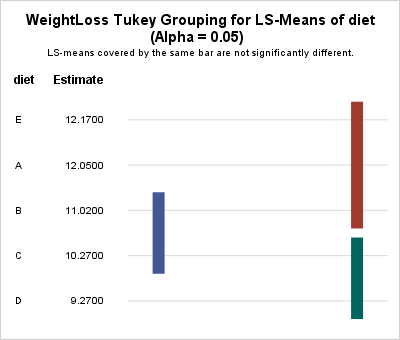How to interpret a lines plot

In the lines plot, the vertical lines visually connect groups whose LS-means are "statistically indistinguishable." Statistically speaking, two means are "statistically indistinguishable" when their pairwise difference is not statistically significant.

If you have k groups, there are k(k-1)/2 pairwise differences that you can examine. The lines plot attempts to summarize those comparisons by connecting groups whose means are statistically indistinguishable. Often there are fewer lines than pairwise comparisons, so the lines plot displays a summary of which groups have similar means.

In the previous analysis, there are five groups, so there are 10 pairwise comparisons of means. The lines plot summarizes the results by using three vertical lines. The leftmost line (blue) indicates that the means of the 'B' and 'C' groups are statistically indistinguishable (they are not significantly different). Similarly, the upper right vertical bar (red) indicates that the means of the pairs ('E','A'), ('E','B'), and ('A','B') are not significantly different from each other. Lastly, the lower right vertical bar (green) indicates that the means for groups 'C' and 'D' are not significantly different. Thus in total, the lines plot indicates that five pairs of means are not significantly different.

The remaining pairs of mean differences (for example, 'E' and 'D') are significantly different. By using only three vertical lines, the lines plot visually associates pairs of means that are essentially the same. Those pairs that are not connected by a vertical line are significantly different.

Advantages and limitations of the lines plot

Advantages of the lines plot include the following:

• The groups are ordered according to the observed means of the groups.
• The number of vertical lines is often much smaller than the number of pairwise comparisons between groups.

Notice that the groups in this example are the same size (10 subjects). When the group sizes are equal (the so-called "balanced ANOVA" case), the lines plot can always correctly represent the relationships between the group means. However, that is not always true for unbalanced data. Westfall et al. (1999, p. 69) provide an example in which using the LINES option on the MEANS statement produces a misleading plot.

The situation is less severe when you use the LSMEANS statement, but for unbalanced data it is sometimes impossible for the lines plot to accurately connect all groups that have insignificant mean differences. In those cases, SAS appends a footnote to the plot that alerts you to the situation and lists the additional significances not represented by the plot.

In my next blog post, I will show some alternative graphical displays that are appropriate for multiple comparisons of means for unbalanced groups.

Summary

In summary, the new lines plot in SAS/STAT software is a graphical version of an analysis that has been in SAS for decades. You can create the plot by using the LINES option in the LSMEANS statement. The lines plot indicates which groups have mean differences that are not significant. For balanced data (or nearly balanced), it does a good job of summarizes which differences of means are not significant. For highly unbalanced data, there are other graphs that you can use. Those graphs will be discussed in a future article.

The post Graphs for multiple comparisons of means: The lines plot appeared first on The DO Loop.Correlations between variables are typically displayed in a matrix. Because the correlation matrix is determined by the order of the variables, it is difficult to find the largest and smallest correlations, which is why analysts sometimes use colors to visualize the correlation matrix. Another visualization option is the pairwise correlation plot, which orders pairs of variables by their correlations.

Neither graph addresses a related problem: for each variable, which other variables are strongly correlated with it? Which are weakly correlated? In SAS, the CORR procedure supports a little-known option that answers that question. You can use the RANK option in the PROC CORR statement to order the correlations for each variable (independently) according to the magnitude of the correlations.

Notice that the RANK option does not compute "rank correlation." You can compute rank correlation by using the SPEARMAN option.

Ordering correlations by size

Consider the Sashelp.Heart data set, which contains data for 5209 patients who enrolled in the Framingham Heart Study. You might want to know which variables are highly correlated with weight, or smoking, or blood pressure, to name a few examples. The following call to PROC CORR uses the RANK option to order each row of correlations in the output:

```/* Note: The MRW variable is similar to the body-mass index (BMI). */ proc corr data=sashelp.Heart RANK noprob; var Height Weight MRW Smoking Diastolic Systolic Cholesterol; run;```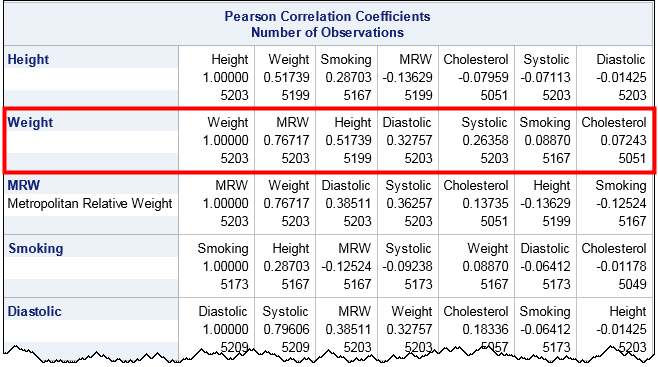The output orders each row according to the magnitude of the correlations. (Click to enlarge.) For example, look at the row for the Weight variable, which is highlighted by a red rectangle. Scanning across the row, you can see that the variables that are the most strongly correlated with Weight are MRW (which measures whether a patient is overweight) and the height. At the end of the row are the variables that are essentially uncorrelated with Weight, namely Smoking and Cholesterol. The numbers at the bottom of each cell indicate the number of nonmissing pairwise observations.

In a similar way, look at the row for the Smoking variable. That variable is most strongly correlated with Height and MRW. Notice that the correlation with MRW is negative, which shows that the correlations are ordered by absolute values (magnitude). The highly correlated variables—whether positively or negatively correlated—appear first and the uncorrelated variable (correlations near zero) occur last in each row.

Ordering correlations for groups of variables

It is common to want to examine the correlations between groups of variables. For example, a clinician might want to look at the correlations between clinical measurements (blood pressure, cholesterol,...) and genetic or lifestyle choices (weight, smoking habits,...). The following call to PROC CORR uses the VAR and WITH statements to compare groups of variables, and uses the RANK option to order the correlations along each row:

```proc corr data=sashelp.Heart RANK noprob; var Height Weight MRW Smoking; /* genetic and lifestyle factors */ with Diastolic Systolic Cholesterol; /* clinical measurements */ run;```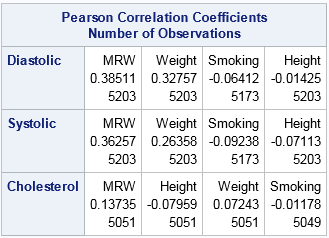Notice that the number of variables in the VAR statement determine the columns. The variables in the WITH statement determine the rows. Within each row, the variables in the VAR statement are ordered by the magnitude of the correlation. For these data, the Diastolic and Systolic variables are similar with respect to how they correlate with the column variables. The order of the column variables is the same for the first two rows. In contrast, the strength of the correlations between the Cholesterol variable and the column variables are in a different order.

In summary, you can use the RANK option in the PROC CORR statement to order the rows of a correlation matrix according to the magnitude (absolute value) of the correlations between each variable and the others. This makes it easy to find pairs of variables that are strongly correlated and pairs that are weakly correlated.

The post Order correlations by magnitude appeared first on The DO Loop.If you perform a weighted statistical analysis, it can be useful to produce a statistical graph that also incorporates the weights. This article shows how to construct and interpret a weighted histogram in SAS.

How to construct a weighted histogram

Before constructing a weighted histogram, let's review the construction of an unweighted histogram. A histogram requires that you specify a set of evenly spaced bins that cover the range of the data. An unweighted histogram of frequencies is constructed by counting the number of observations that are in each bin. Because counts are dependent on the sample size, n, histograms often display the proportion (or percentage) of values in each bin. The proportions are the counts divided by n. On the proportion scale, the height of each bin is the sum of the quantity 1/n, where the sum is taken over all observations in the bin.

That fact is important because it reveals that the unweighted histogram is a special case of the weighted histogram. An unweighted histogram is equivalent to a weighted histogram in which each observation receives a unit weight. Therefore the quantity 1/n is the standardized weight of each observation: the weight divided by the sum of the weights. The formula is the same for non-unit weights: the height of each bin is the sum of the quantity wi / Σ wi, where the sum is taken over all observations in the bin. That is, you add up all the standardized weights in each bin to produce the bin height.

An example of a weighted histogram

The SAS documentation for the WEIGHT statement includes the following example. Twenty subjects estimate the diameter of an object that is 30 cm across. Some people are placed closer to the object than others. The researcher believes that the precision of the estimate is inversely proportional to the distance from the object. Therefore the researcher weights each subject's estimate by using the inverse distance.

The following DATA step creates the data, and PROC SGPLOT creates a weighted histogram of the data by using the WEIGHT= option on the HISTOGRAM option. (The WEIGHT= option was added in SAS 9.4M1.)

```data Size; input Distance ObjectSize @@; Wt = 1 / distance; /* precision */ x = ObjectSize; label x = "Estimate of Size"; datalines; 1.5 30 1.5 20 1.5 30 1.5 25 3 43 3 33 3 25 3 30 4.5 25 4.5 36 4.5 48 4.5 33 6 43 6 36 6 23 6 48 7.5 30 7.5 25 7.5 50 7.5 38 ;   title "Weighted Histogram of Size Estimate"; proc sgplot data=size noautolegend; histogram x / WEIGHT=Wt scale=proportion datalabel binwidth=5; fringe x / lineattrs=(thickness=2 color=black) transparency=0.6; yaxis grid offsetmin=0.05 label="Weighted Proportion"; refline 30 / axis=x lineattrs=(pattern=dash); run;```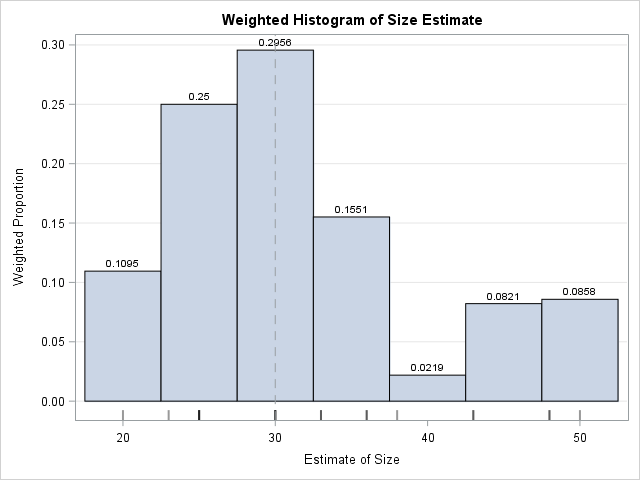The weighted histogram is shown to the right. The data values are shown in the fringe plot beneath the histogram. The height of each bin is the sum of the weights of the observations in that bin. The dashed line represents the true diameter of the object. Most estimates are clustered around the true value, except for a small cluster of larger estimates. Notice that I use the SCALE=PROPORTION option to plot the weighted proportion of observations in each bin, although the default behavior (SCALE=PERCENT) would also be acceptable.

If you remove the WEIGHT= option and study the unweighted graph, you will see that the average estimate for the unweighted distribution (33.6) is not as close to the true diameter as the weighted estimate (30.1). Furthermore, the weighted standard deviation is about half the unweighted standard deviation, which shows that the weighted distribution of these data has less variance than the unweighted distribution.

By the way, although PROC UNIVARIATE can produce weighted statistics, it does not create weighted graphics as of SAS 9.4M5. One reason is that the graphics statements (CDFPLOT, HISTOGRAM, QQPLOT, etc) not only create graphs but also fit distributions and produce goodness-of-fit statistics, and those analyses do not support weight variables.

Checking the computation

Although a weighted histogram is not conceptually complex, I understand a computation better when I program it myself. You can write a SAS program that computes a weighted histogram by using the following algorithm:

1. Construct the bins. For this example, there are eight bins of width 5, and the first bin starts at x=17.5. (It is centered at x=20.) Initialize all bin heights to zero.
2. For each observation, find the bin that contains it. Increment the bin height by the weight of that observation.
3. Standardize the heights by dividing by the sum of weights. You can skip this step if the weights sum to unity.

A SAS/IML implementation of this algorithm requires only a few lines of code. A DATA step implementation that uses arrays is longer, but probably looks more familiar to many SAS programmers:

```data BinHeights(keep=height:); array EndPt _temporary_; binStart = 17.5; binWidth = 5; /* anchor and width for bins */ do i = 1 to dim(EndPt); /* define endpoints of bins */ EndPt[i] = binStart + (i-1)*binWidth; end;   array height; /* height of each bin */ set Size end=eof; /* for each observation ... */ sumWt + Wt; /* compute sum of weights */ Found=0; do i = 1 to dim(EndPt)-1 while (^Found); /* find bin for each obs */ Found = (EndPt[i] <= x < EndPt[i+1]); if Found then height[i] + Wt; /* increment bin height by weight */ end; if eof then do; do i = 1 to dim(height); /* scale heights by sum of weights */ height[i] = height[i] / sumWt; end; output; end; run;   proc print noobs data=BinHeights; run;```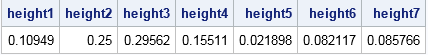The computations from the DATA step match the data labels that appear on the weighted histogram in PROC SGPLOT.

Summary

In SAS, the HISTOGRAM statement in PROC SGPLOT supports the WEIGHT= option, which enables you to create a weighted histogram. A weighted histogram shows the weighted distribution of the data. If the histogram displays proportions (rather than raw counts), then the heights of the bars are the sum of the standardized weights of the observations within each bin. You can download the SAS program that computes the quantities in this article.

How can you interpret a weighted histogram? That depends on the meaning of the weight variables. For survey data and sampling weights, the weighted histogram estimates the distribution of a quantity in the population. For inverse variance weights (such as were used in this article), the weighted histogram overweights precise measurements and underweights imprecise measurements. When the weights are correct, the weighted histogram is a better estimate of the density of the underlying population and the weighted statistics (mean, variance, quantiles,...) are better estimates of the corresponding population quantities.